# Outline

• Simple plotting using default graphics tools in R

• Plotting with graphic packages in R (ggplot2)

• Visualizing data by different types of graphs in R
• Scatter plot, line graph, bar graph, histogram, boxplot, pie chart, Venn diagram, correlation plot, heatmap
• Generate polished graph for publication and presentation

# Why using R for plotting

• When the large sample size exceed the capacity for Excel, Prism or other graphic tools

• Fast and simple

• Bundle with complicated statistical analysis

• Strong graphics capabilities makes R more popular

• Many add-on packages for various needs

# Basic plot example

``````library("datasets")
data()
str(pressure)``````
``````'data.frame':   19 obs. of  2 variables:
\$ temperature: num  0 20 40 60 80 100 120 140 160 180 ...
\$ pressure   : num  0.0002 0.0012 0.006 0.03 0.09 0.27 0.75 1.85 4.2 8.8 ...``````
``````View(pressure)
plot(pressure)``````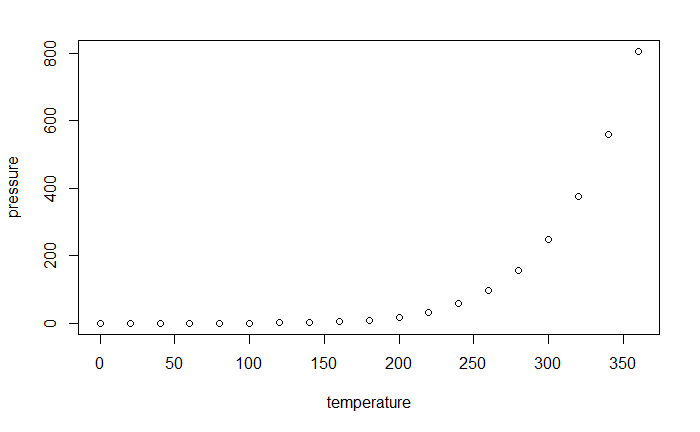# Labels and Axes

• Default: R uses the variable names for axes labels and computes range for axes.

• Manual change by:
• axes labels: xlab, ylab
• size of labels: cex.lab
• axes range: xlim, ylim

# Titles

• main: sets plot title (above plot)

• sub: sets subtitle (beneath plot)

# Symbols, colors, and lines

• type: “p” for points, “l” for lines, “b” for both, “h” for histogram-like

• pch: point symbol

• col: color

• cex: size factor

• lty: line type

• lwd: line width

# Plot symbols

#### Use the pch= option to specify symbols to use when plotting points. For symbols 21 through 25, specify border color (col=) and fill color (bg=).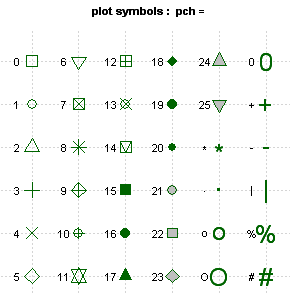# Default color palette in R

``````palette("default") # you'll only need this line if you've previously changed the palette from the default
palette()``````
`` "black"   "red"     "green3"  "blue"    "cyan"    "magenta" "yellow"  "gray"   ``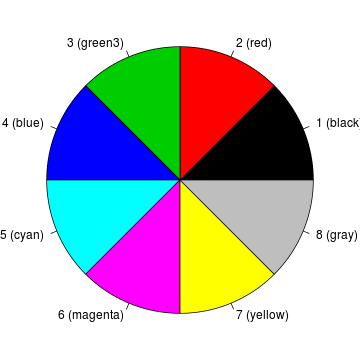• Other R color palette options
• rainbow(n)
• heat.colors(n)
• terrain.colors(n)
• topo.colors(n)
• cm.colors(n)
• RColorBrewer package palette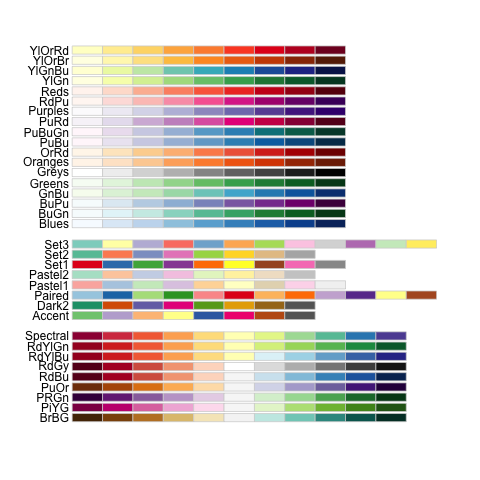# Line types (lty= )``````par(mfrow=c(1,3))
#Solid line (by default)
plot(1:10, 1:10, type="l")
#Use dashed line type
plot(1:10, 1:10, type="l", lty=2)``````
``````#Change line width
plot(1:10, 1:10, type="l", lty=2, lwd=3)``````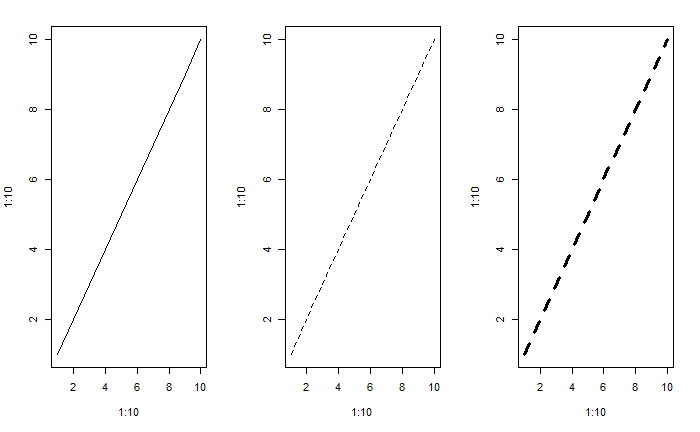# Example: plot points with formatting

``plot(pressure, type="p")````plot(pressure, type="p", pch=8, cex=0.8, col="red")``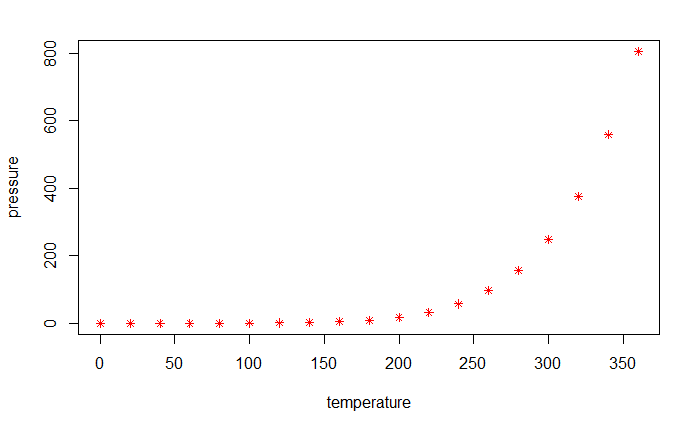# Example: Line graph with formatting

``plot(pressure, type="l", lty=3, lwd=2, col="blue")``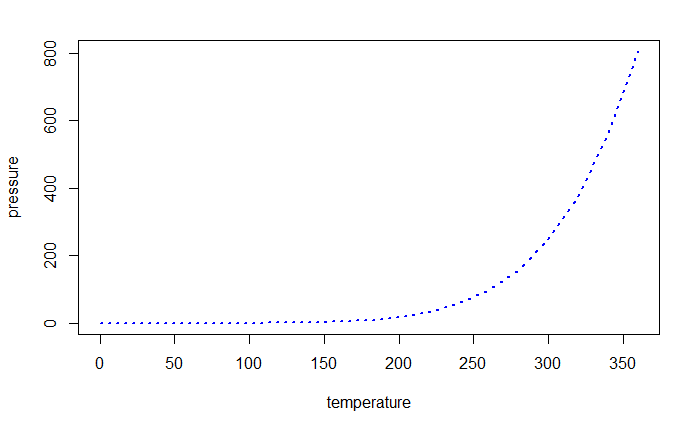# Example: Add title and text

``plot(pressure, main="Relation")``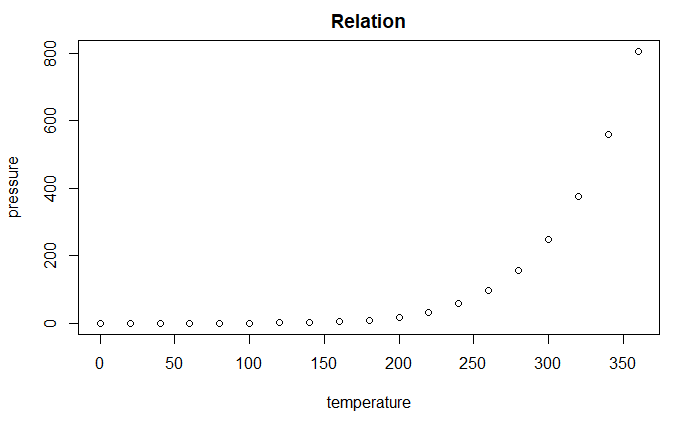``````plot(pressure)
text(150, 200, label="p-value = 0.05")``````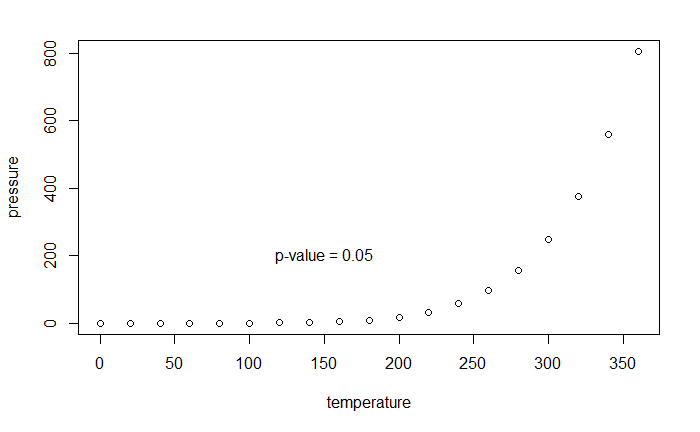# Plot for multiple groups

``````data(iris) #load iris data
pch.vec <- c(2,8,21)[iris\$Species]
col.vec <- c(2,3,6)[iris\$Species]
plot(iris\$Sepal.Length, iris\$Sepal.Width, col=col.vec, pch=pch.vec, xlab="sepal.length", ylab="sepal.width", main="iris")
legend("topleft", pch=c(2,8,21), col=c(2,3,6), legend=unique(iris\$Species), cex=0.8)``````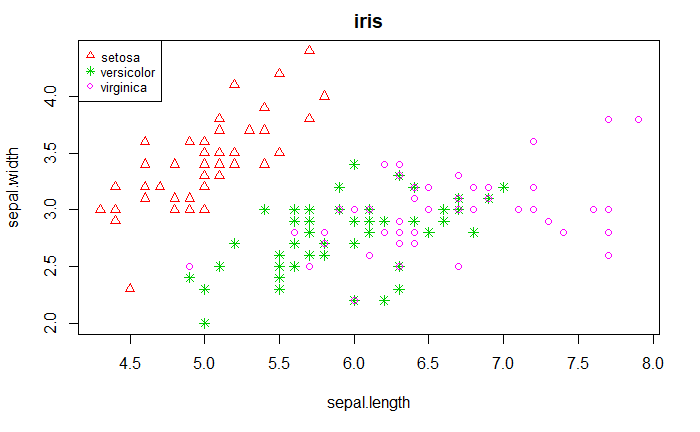# Beyond simple graphs: ggplot2

• Hadley Wickham’s ggplots package provides a unified interface and simple set of options.

• Once you understand how ggplots works for one type of plot, you can easily apply the knowledge for any other types of plots.

• It provides beautiful and publication-ready results.

• Easy to plot for data with multiple groups and build legend automatically.

# Build-in R plotting vs. ggplot2

``library(ggplot2)``
``Need help? Try Stackoverflow: https://stackoverflow.com/tags/ggplot2.``
``````#View(diamonds)
plot(diamonds\$carat, diamonds\$price, col=diamonds\$color, pch=as.numeric(diamonds\$cut))``````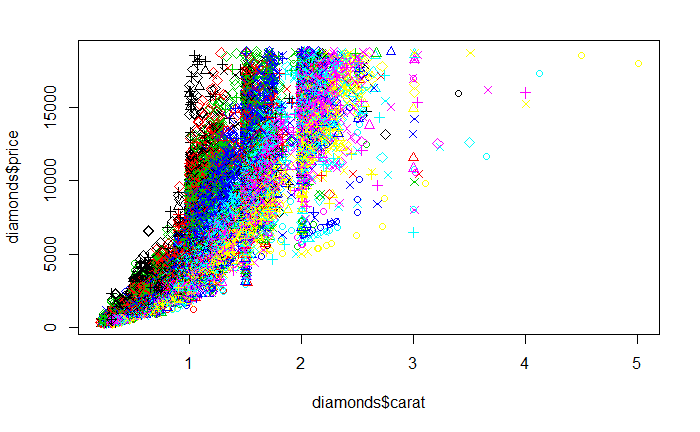``ggplot(diamonds, aes(carat, price, col=color, shape=cut)) + geom_point()``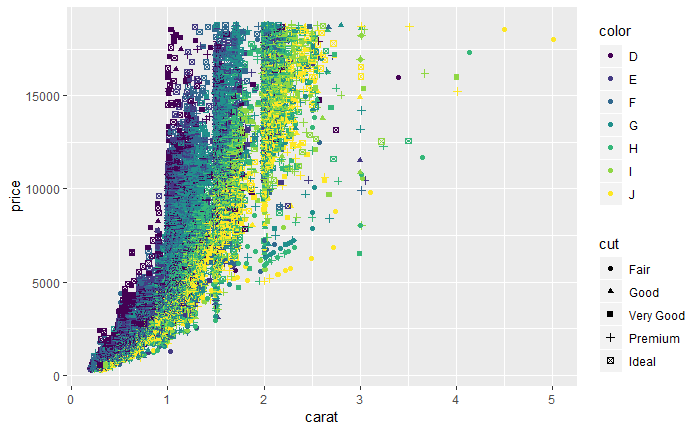# Scatter plot

• Display the relationship between two continuous variables
``ggplot(iris, aes(x=Sepal.Length, y=Sepal.Width, shape=Species, colour=Species)) + geom_point()``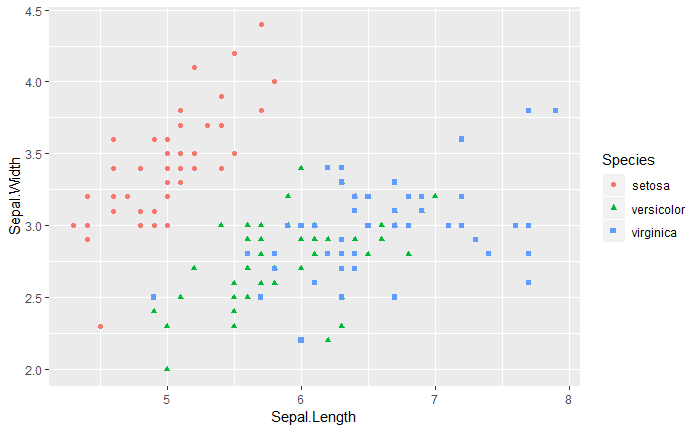``````ggplot(iris, aes(x=Sepal.Length, y=Sepal.Width, shape=Species, colour=Species)) + geom_point() +
scale_color_brewer(palette="Dark2") +
scale_shape_manual(values=c(2,8,0)) +
labs(x="Length", y="Width", title="Sepal Length vs. Width") +
theme(plot.title = element_text(hjust=0.5))``````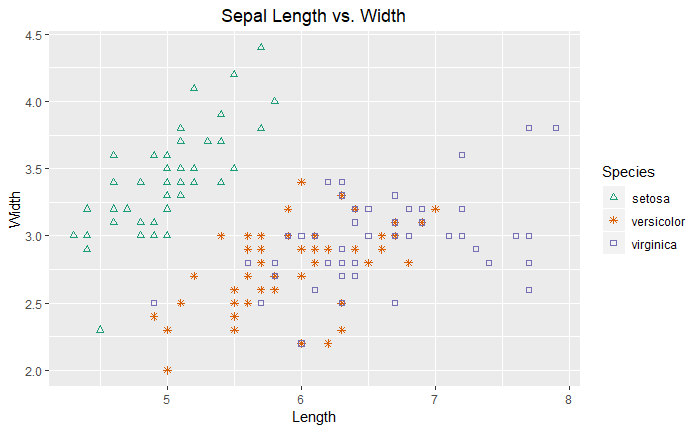``````ggplot(iris, aes(x=Sepal.Length, y=Sepal.Width, shape=Species, colour=Species)) + geom_point() +
geom_smooth(method=lm,, se=FALSE)``````